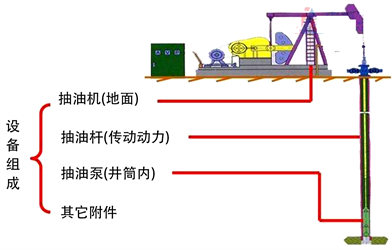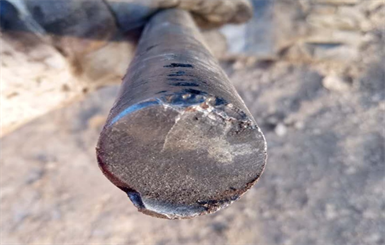#### 期刊菜单

Analysis of Sucker Rod Corrosion Mechanism and Research and Application of New Anticorrosion Technology in Shengtuo Oilfield
DOI: 10.12677/JOGT.2021.434074, PDF , HTML, XML, 下载: 269  浏览: 336

Abstract: Through a brief analysis of the sucker rod corrosion phenomenon during the 50-year develop-ment of Shengtuo Oilfield, the reasons leading to the failure of the sucker rod are summarized. In view of the current situation of sucker rod corrosion, the application of new continuous sucker rods with anti-corrosion ability, the application of new stainless steel weighting rod balancing technology, the application of wellhead corrosion inhibitor technology and IPR curve analysis technology are proposed to reduce the corrosion of sucker rods and resist corrosion. Both corrosion resistance and fatigue resistance have been greatly improved, and good results have been achieved after field application, which provides a good reference for the anti-corrosion technology of sucker rods in late-stage oilfields with ultra-high water cut.

1. 引言Figure 1. Pumping machine system diagram

2. 胜坨油田抽油杆腐蚀原因分析

2.1. CO2影响

2.1.1. 全面腐蚀

2.1.2. 局部腐蚀Figure 2. Worm effect invalid photo

2.2. H2S的影响

H2S腐蚀坑是随机排列的，呈圆锥形，侧面较陡，坑的边缘圆滑，坑之间不相连，腐蚀物呈黑色较粘，有硫化氢味。

2.3. 氧腐蚀

$\text{Fe}\to {\text{Fe}}^{\text{2}}+{\text{2e}}^{-}$ (1)

${\text{O}}^{\text{2}}+{\text{2H}}_{\text{2}}\text{O}+{\text{4e}}^{-}\to {\text{4OH}}^{-}$ (2)

${\text{Fe}}^{\text{2}+}+{\text{2OH}}^{-}\to \text{Fe}{\left(\text{OH}\right)}_{\text{2}}$ (3)

$\text{4Fe}{\left(\text{OH}\right)}_{\text{2}}+{\text{2H}}_{\text{2}}\text{O}+{\text{O}}_{\text{2}}\to \text{4Fe}{\left(\text{OH}\right)}_{\text{3}}$ (4)

$\text{2Fe}{\left(\text{OH}\right)}_{\text{3}}-{\text{H}}_{\text{2}}\text{O}\to {\text{Fe}}_{\text{2}}{\text{O}}_{\text{3}}\cdot {\text{2H}}_{2}\text{O}$ (5)

$\text{Fe}{\left(\text{OH}\right)}_{\text{3}}-{\text{H}}_{\text{2}}\text{O}\to \text{FeO}\left(\text{OH}\right)$ (6)

$\text{8FeO}{\left(\text{OH}\right)}_{\text{3}}+{\text{Fe}}^{\text{2}+}+{\text{2e}}^{-}\to {\text{3Fe}}_{\text{3}}{\text{O}}_{\text{4}}+{\text{4H}}_{\text{2}}\text{O}$ (7)

2.4. 其他腐蚀因素

3. 胜坨油田抽油杆防腐技术研究

3.1. 应用具有防腐能力的抽油杆

3.2. 应用井口加缓蚀剂技术

3.3. 应用IPR曲线系统沉没度优化技术

${H}_{液面}={H}_{油层}-\left[\left({P}_{i}-\frac{Q}{J}\right)÷9.8×100-300{\rho }_{o}\right]÷{\rho }_{l}-300$ (8)

H液面——油井动液面(m)

H油层——油层垂深(m)

Pi——地层静压(MPa)

Q——油井日液(t)

J——采液指数(t/MPa∙d)

ρo——原油密度(g/cm3)

${\rho }_{l}$ ——混合液密度(g/cm3)

4. 结论

1) 高含水工况下胜坨油田抽油杆失效原因主要有CO2腐蚀、H2S腐蚀、氧腐蚀以及综合腐蚀。

2) 使用φ28 mm和φ32 mm的高强抽油杆，满足大直径抽油泵，对抽油杆的抗拉强度提出了新的要求，能够有效延长抽油杆使用寿命。

3) 应用井口加缓蚀剂技术，在管、杆表面形成一完整的保护膜来抑制腐蚀，可以更好地适应高含水、高液量的恶劣工作环境。

4) 运用IPR曲线系统沉没度优化技术，实现单井单策的工况优化方法，进一步提高腐蚀疲劳寿命。

  W. B. 斯蒂瓦德, 袁克勤. 抽油杆的失效[J]. 石油机械, 1980(3): 44.  边勇俊, 石伟, 劳金越, 等. 20CrMo钢抽油杆断裂原因分析[J]. 金属热处理, 2011, 36(9): 106-108.  刘婉. 油田缓蚀剂的分类及机理探讨[J]. 化工技术与开发, 2018, 47(3): 29-33.  王建军, 付太森, 薛承文, 孙建华, 李方坡. 地下储气库套管和油管腐蚀选材分析[J]. 石油机械, 2017, 45(1): 110-113.  孙喆. 胜坨油田抽油杆失效机理分析及新型防腐抽油杆应用研究[D]: [硕士学位论文]. 青岛: 中国石油大学(华东), 2018: 3-5.  穆哈拜, 杨新明, 户贵华, 李素芹, 勒从起, 张晓梅. 油井管杆牺牲阳极防腐蚀装置的研制与应用[J]. 腐蚀与防护, 2000, 21(7): 310-312.  蔡锐, 赵金龙, 吴鹏, 张哲. L80油管螺纹接头腐蚀原因分析[J]. 理化检验: 物理分册, 2019, 55(4): 278-281.  赵永峰. 13Cr钢在CO2-H2S-CL体系中的腐蚀及其缓蚀技术研究[D]: [博士学位论文]. 北京: 北京化工大学, 2010: 10-20.  Sirgo, E.C., Gibson, E.D. and Jackson, W.E. (1998) Polyethylene Lined Tubing in Rod Pumped Wells. SPE Permian Basin Oil and Gas Recovery Conference, Midland, Texas, March 1998, SPE-39815-MS. https://doi.org/10.2118/39815-MS  杨圣贤, 严科, 段建辉, 赵国芳. 基于阶段生产数据的IPR曲线类型及意义[J]. 特种油气藏, 2017, 24(1): 92-95.  迟阿惠. 抽油杆防腐技术探讨[J]. 中国化工装备, 2020(1): 41-44.